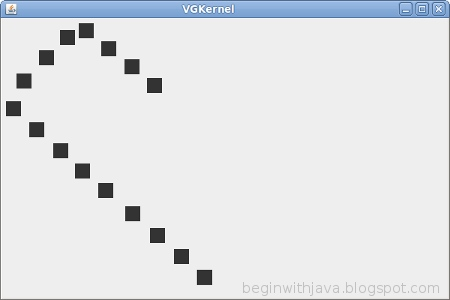## Wednesday, August 4, 2010

### A Simple Java Video Game Kernel

Most video games run continuously, rather than waiting for user input before they do something. The heart of a video game of this sort is called the kernel. The kernel is running in the background, collecting user input and updating the display. It uses logic to determine if the game has been won or lost or otherwise control the state of the game.##### This simple kernel sends a ball bouncing around on the screen. Turn it into your own Pong, Breakout, or Tank clone.

To run this way, Threads are usually used to allow more than one thing to be going on at a time in a Java program. We've looked at a simple way of using threads before, the Timer class.

Here's a really, really simple video game kernel. It has all the basic elements of a video game.
```/* A simple video game style kernel
by Mark Graybill, August 2010
Uses the Timer Class to move a ball on a playfield.
*/

// Import Timer and other useful stuff:
import java.util.*;
// Import the basic graphics classes.
import java.awt.*;
import javax.swing.*;

public class VGKernel extends JPanel{

// Set up the objects and variables we'll want.
public Rectangle screen, ball; // The screen area and ball location/size.
public Rectangle bounds;  // The boundaries of the drawing area.
public JFrame frame; // A JFrame to put the graphics into.
public boolean down, right; // Direction of ball's travel.

// Create a constructor method that initializes things:
public VGKernel(){
super();
screen = new Rectangle(0, 0, 600, 400);
ball   = new Rectangle(0, 0, 20, 20);
bounds = new Rectangle(0, 0, 600, 400); // Give some starter values.
frame = new JFrame("VGKernel");
}
// members of the VGKernel.
public void run(){
moveBall();
frame.repaint();
}
}

// Now the instance methods:
public void paintComponent(Graphics g){
// Get the drawing area bounds for game logic.
bounds = g.getClipBounds();
// Clear the drawing area, then draw the ball.
g.clearRect(screen.x, screen.y, screen.width, screen.height);
g.fillRect(ball.x, ball.y, ball.width, ball.height);
}

public void moveBall(){
// Ball should really be its own class with this as a method.
if (right) ball.x+=ball.width; // If right is true, move ball right,
else ball.x-=ball.width;       // otherwise move left.
if (down)  ball.y+=ball.height; // Same for up/down.
else ball.y-=ball.width;
if (ball.x > (bounds.width - ball.width)) // Detect edges and bounce.
{ right = false; ball.x = bounds.width -  ball.width; }
if (ball.y > (bounds.height - ball.height))
{ down  = false; ball.y = bounds.height - ball.height;}
if (ball.x <= 0) { right = true; ball.x = 0; }
if (ball.y <= 0) { down  = true; ball.y = 0; }
}

public static void main(String arg[]){
java.util.Timer vgTimer = new java.util.Timer();  // Create a Timer.
VGKernel panel = new VGKernel(); // Create and instance of our kernel.

// Set the intial ball movement direction.
panel.down = true;
panel.right = true;

// Set up our JFRame
panel.frame.setDefaultCloseOperation(JFrame.EXIT_ON_CLOSE);
panel.frame.setSize(panel.screen.width, panel.screen.height);
panel.frame.setContentPane(panel);
panel.frame.setVisible(true);

// Set up a timer to do the vgTask regularly.
}
}```

This example can be expanded with methods to get control inputs, additional players on the playfield (like paddles), and logic to determine when someone scores.

The code here is far from perfect, but I've made some compromises to make things as simple as I could while still showing a full working example. Not that any code that runs and does what is supposed to is really bad, but there are other, better ways of doing this. But this works and is fairly easy to understand.

What the program does is create a JPanel that has an inner class (a class defined within itself) of VGTimerTask. The VGTimerTask is a kind of TimerTask, which can be scheduled to occur on a regular basis by a Timer. Since VGTimerTask is an inner class of VGPanel, it has access to all the members of VGPanel. This is critical. Without that, it wouldn't be able to access the ball and redraw the screen easily (it can still be done, but in a more complex way.)

Timer is a decent way of running a simple game, but more complex games should use some other timing mechanism. java.util.Timer is affected by a number of outside events, so to get smoother, more reliable timing you a timer like the one in the Java3D package would work better.

A Simple Improvement

There are many ways of improving on this basic example. One way that is very simple is to smooth the animation. The movement of the ball is pretty jerky. This is caused by both the distance that the ball moves each "turn", and by the time between screen updates. We can smooth out the animation by addressing both of these.

First, let's change moveBall() to shift the ball a smaller distance each time:
```public void moveBall(){
// Ball should really be its own class with this as a method.
if (right) ball.x+=ball.width/4; // If right is true, move ball right,
else ball.x-=ball.width/4;       // otherwise move left.
if (down)  ball.y+=ball.height/4; // Same for up/down.
else ball.y-=ball.width/4;
if (ball.x > (bounds.width - ball.width)) // Detect edges and bounce.
{ right = false; ball.x = bounds.width -  ball.width; }
if (ball.y > (bounds.height - ball.height))
{ down  = false; ball.y = bounds.height - ball.height;}
if (ball.x <= 0) { right = true; ball.x = 0; }
if (ball.y <= 0) { down  = true; ball.y = 0; }
}```
Now the ball is being moved only one quarter of its size each turn.

Next, change the Timer schedule to draw the screen every 20 milliseconds instead of every 100 milliseconds:
```// Set up a timer to do the vgTask regularly.